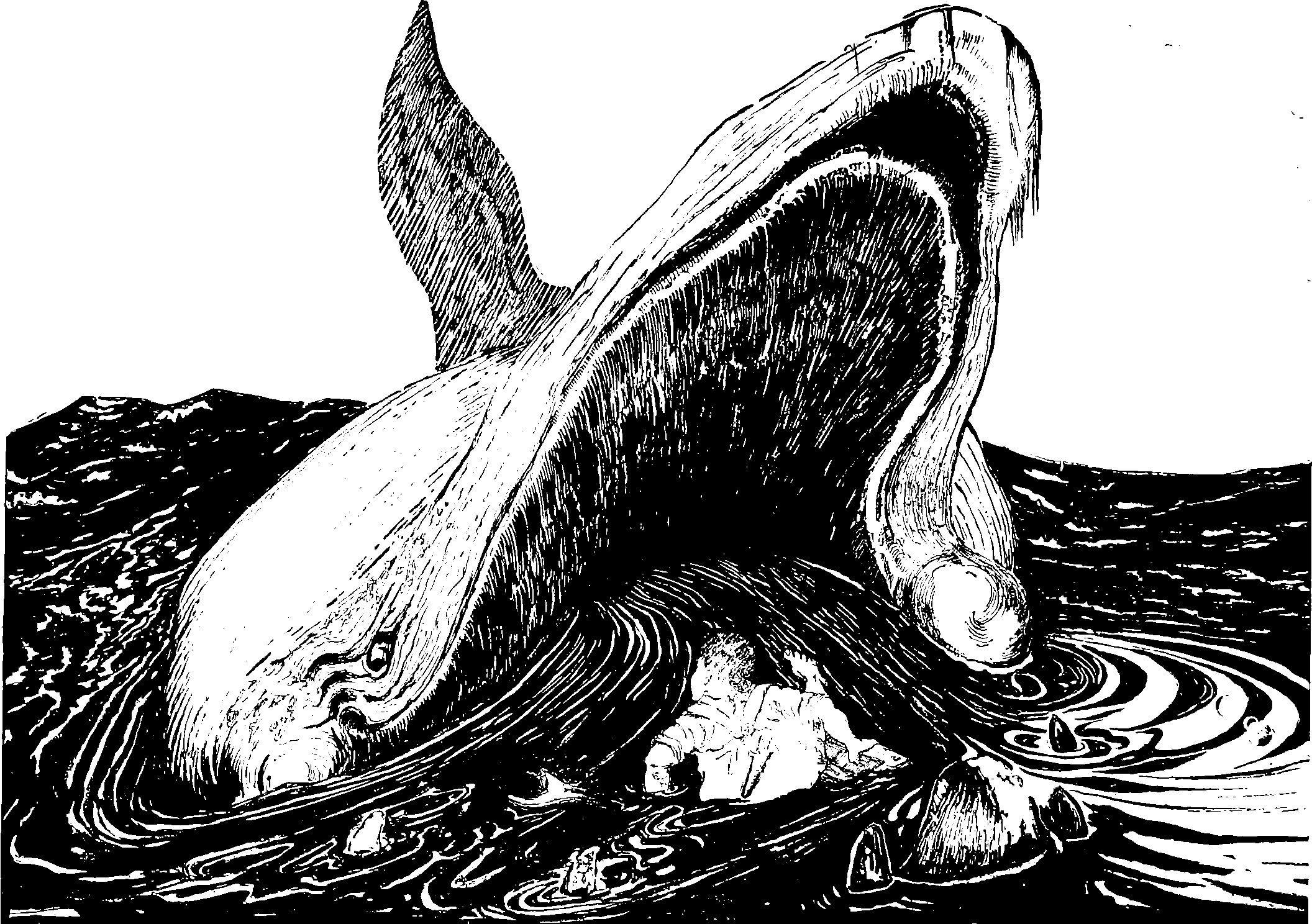# Infinite width limits of neural networksLarge-width limits of neural nets

## Neural Network Gaussian Process

The field that sprang from the insight that in the infinite limit deep NNs asymptotically approach Gaussian processes, and there are theories we can draw from that. Far from the infinite limit there are neural nets which exploit this. See random neural nets.

## Neural Network Tangent KernelFerenc Huszár provides some Intuition on the Neural Tangent Kernel, i.e. the paper .

It turns out the neural tangent kernel becomes particularly useful when studying learning dynamics in infinitely wide feed-forward neural networks. Why? Because in this limit, two things happen:

1. First: if we initialize $$θ_0$$ randomly from appropriately chosen distributions, the initial NTK of the network $$k_{θ_0}$$ approaches a deterministic kernel as the width increases. This means, that at initialization, $$k_{θ_0}$$ doesn’t really depend on $$k_{θ_0}$$ but is a fixed kernel independent of the specific initialization.-
2. Second: in the infinite limit the kernel $$k_{θ_t}$$ stays constant over time as we optimise $$\theta_t$$. This removes the parameter dependence during training.

These two facts put together imply that gradient descent in the infinitely wide and infinitesimally small learning rate limit can be understood as a pretty simple algorithm called kernel gradient descent with a fixed kernel function that depends only on the architecture (number of layers, activations, etc).

These results, taken together with an older known result by Neal, (1994), allows us to characterise the probability distribution of minima that gradient descent converges to in this infinite limit as a Gaussian process.

## Implicit regularization

Here’s one interesting perspective .

• The effective capacity of neural networks is large enough for a brute-force memorization of the entire data set.

• Even optimization on random labels remains easy. In fact, training time increases only by a small constant factor compared with training on the true labels.

• Randomizing labels is solely a data transformation, leaving all other properties of the learning problem unchanged.

[…] Explicit regularization may improve generalization performance, but is neither necessary nor by itself sufficient for controlling generalization error. […] Appealing to linear models, we analyze how SGD acts as an implicit regularizer.

### Dropout

turns out to be a type of regularization with a natural GP interpretation.